# TwoVariable Regression Model The problem of Estimation Unit

• Slides: 25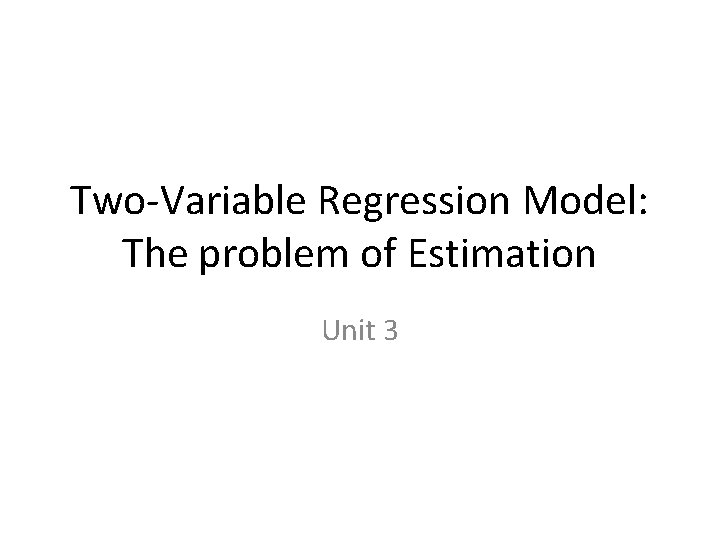Two-Variable Regression Model: The problem of Estimation Unit 3We will cover the following: 1. Method of ordinary least square (OLS) 2. Estimation of OLS regression parameters 3. Classical linear regression model: Assumptions underlying the model of OLS 4. Properties of least square estimators: Gauss. Markov Theorem 5. Coefficient of determinationThe method of OLS The method of ordinary least squares is attributed to Carl Friedrich Gauss, a German mathematician. Under certain assumptions which we will discuss later, the method of least squares has some very attractive statistical properties that have made it one of the most powerful and popular methods of regression analysis. To understand this method, we first explain the least squares principle.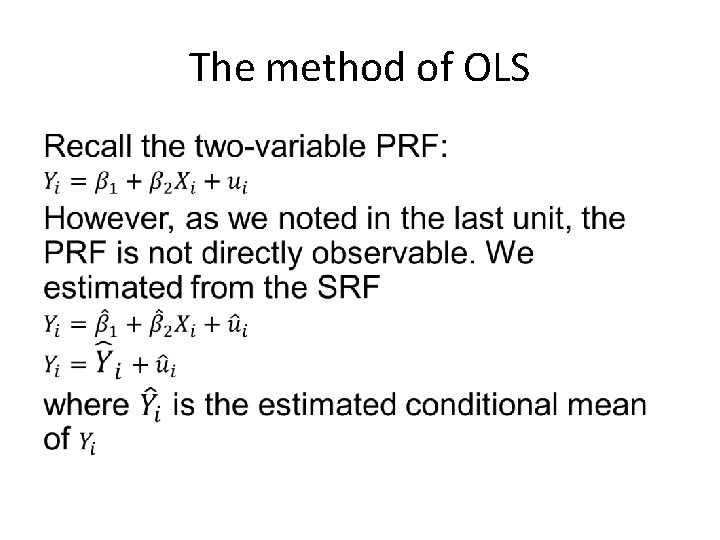The method of OLS •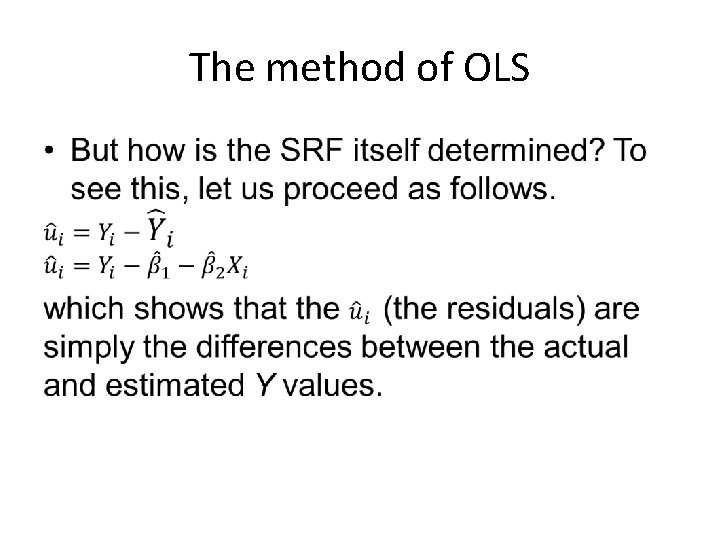The method of OLS •The method of OLS •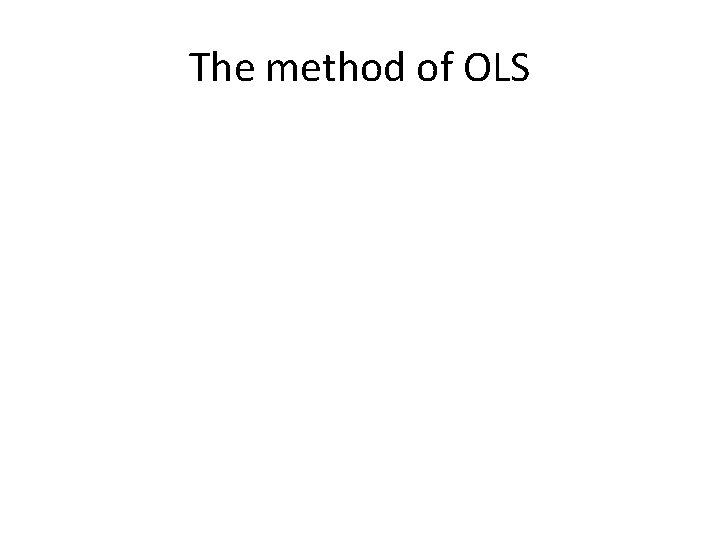The method of OLS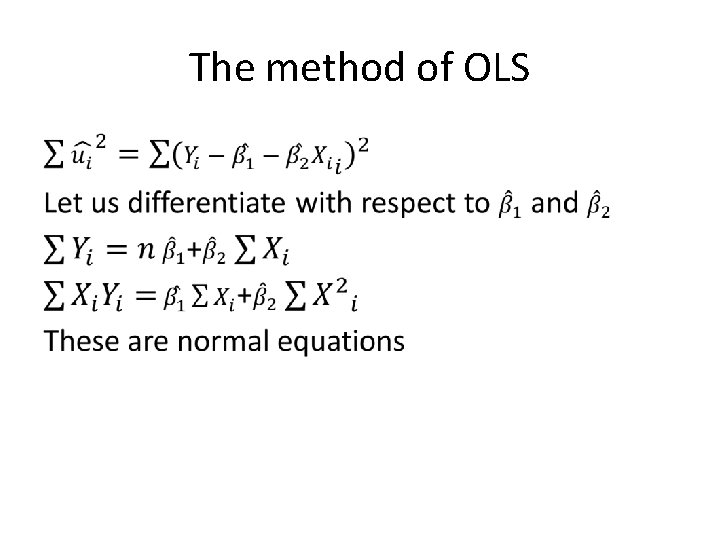The method of OLS •The Classical Linear Regression Model: The Assumptions underlying the method of least square •The Classical Linear Regression Model: The Assumptions underlying the method of least square To see why this requirement is needed, look at the PRF: Yi = β 1 + β 2 Xi + ui. It shows that Yi depends on both Xi and ui. Therefore, unless we are speciﬁc about how Xi and ui are created or generated, there is no way we can make any statistical inference about the Yi and also, as we shall see, about β 1 and β 2. Thus, the assumptions made about the Xi variable(s) and the error term are extremely critical to the valid interpretation of the regression estimates.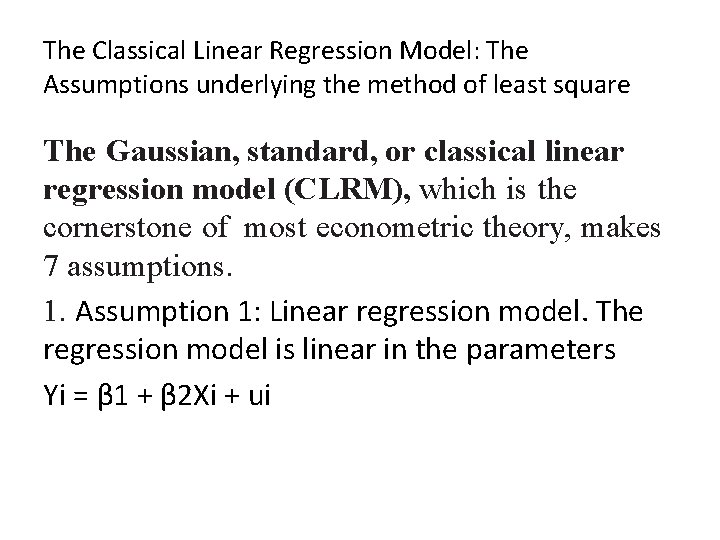The Classical Linear Regression Model: The Assumptions underlying the method of least square The Gaussian, standard, or classical linear regression model (CLRM), which is the cornerstone of most econometric theory, makes 7 assumptions. 1. Assumption 1: Linear regression model. The regression model is linear in the parameters Yi = β 1 + β 2 Xi + ui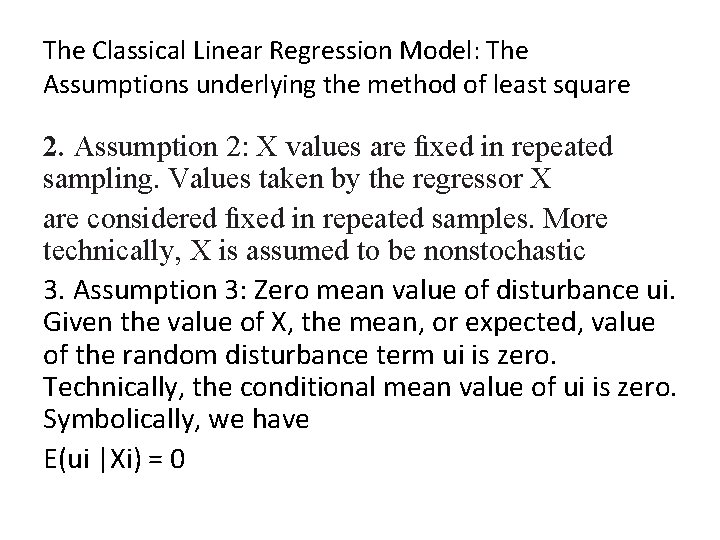The Classical Linear Regression Model: The Assumptions underlying the method of least square 2. Assumption 2: X values are ﬁxed in repeated sampling. Values taken by the regressor X are considered ﬁxed in repeated samples. More technically, X is assumed to be nonstochastic 3. Assumption 3: Zero mean value of disturbance ui. Given the value of X, the mean, or expected, value of the random disturbance term ui is zero. Technically, the conditional mean value of ui is zero. Symbolically, we have E(ui |Xi) = 0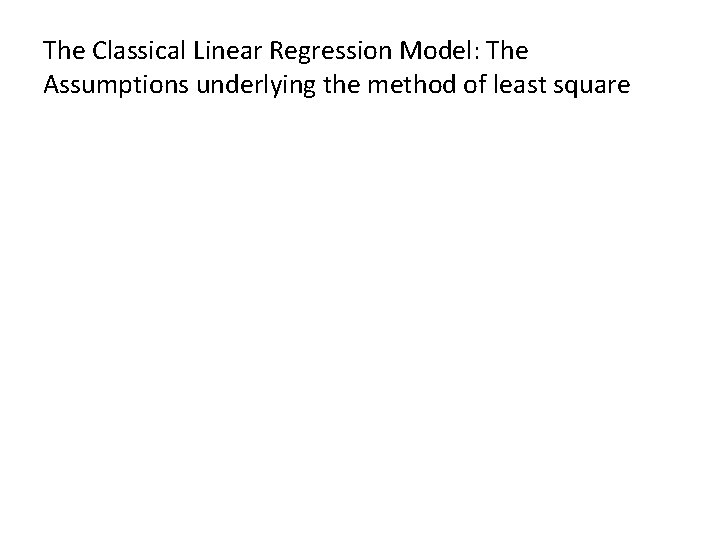The Classical Linear Regression Model: The Assumptions underlying the method of least squareThe Classical Linear Regression Model: The Assumptions underlying the method of least square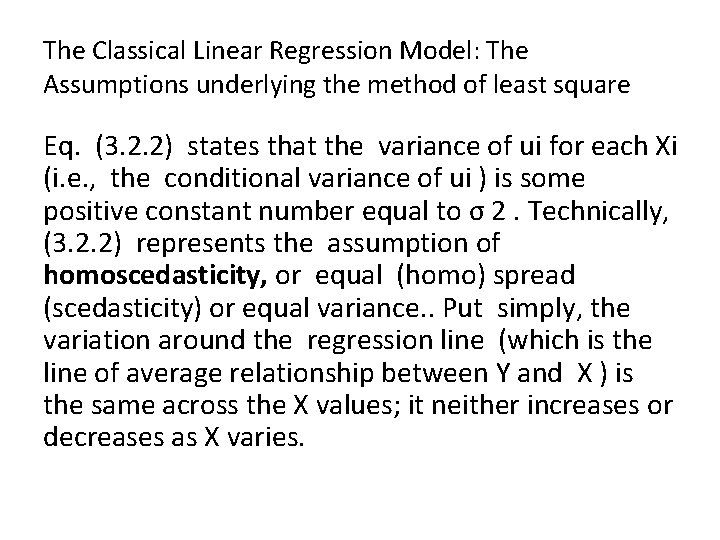The Classical Linear Regression Model: The Assumptions underlying the method of least square Eq. (3. 2. 2) states that the variance of ui for each Xi (i. e. , the conditional variance of ui ) is some positive constant number equal to σ 2. Technically, (3. 2. 2) represents the assumption of homoscedasticity, or equal (homo) spread (scedasticity) or equal variance. . Put simply, the variation around the regression line (which is the line of average relationship between Y and X ) is the same across the X values; it neither increases or decreases as X varies.The Classical Linear Regression Model: The Assumptions underlying the method of least square Diagrammatically, the situation is as depicted in Figure 3. 4.The Classical Linear Regression Model: The Assumptions underlying the method of least squareThe Classical Linear Regression Model: The Assumptions underlying the method of least square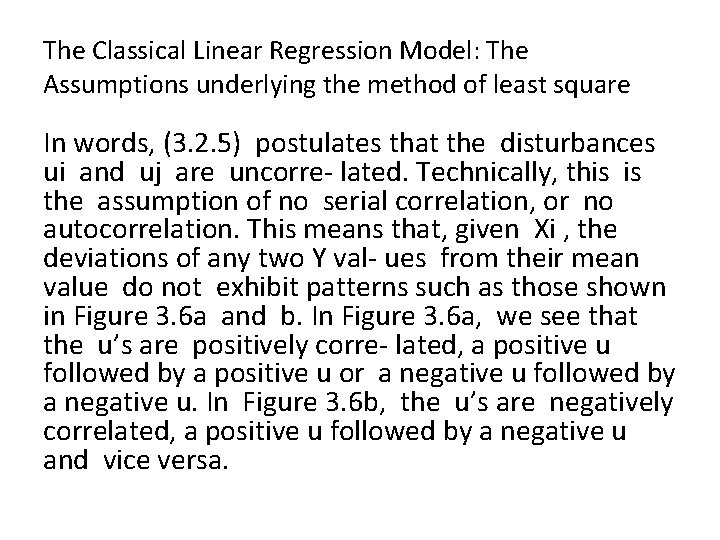The Classical Linear Regression Model: The Assumptions underlying the method of least square In words, (3. 2. 5) postulates that the disturbances ui and uj are uncorre- lated. Technically, this is the assumption of no serial correlation, or no autocorrelation. This means that, given Xi , the deviations of any two Y val- ues from their mean value do not exhibit patterns such as those shown in Figure 3. 6 a and b. In Figure 3. 6 a, we see that the u’s are positively corre- lated, a positive u followed by a positive u or a negative u followed by a negative u. In Figure 3. 6 b, the u’s are negatively correlated, a positive u followed by a negative u and vice versa.The Classical Linear Regression Model: The Assumptions underlying the method of least square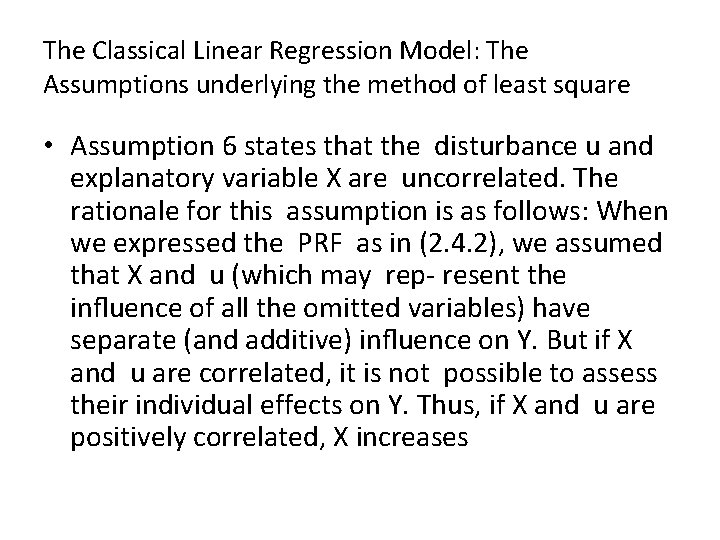The Classical Linear Regression Model: The Assumptions underlying the method of least square • Assumption 6 states that the disturbance u and explanatory variable X are uncorrelated. The rationale for this assumption is as follows: When we expressed the PRF as in (2. 4. 2), we assumed that X and u (which may rep- resent the inﬂuence of all the omitted variables) have separate (and additive) inﬂuence on Y. But if X and u are correlated, it is not possible to assess their individual effects on Y. Thus, if X and u are positively correlated, X increases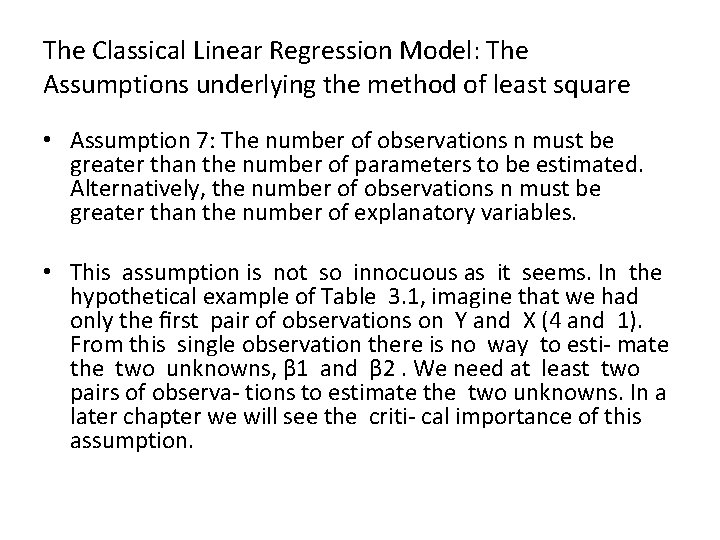The Classical Linear Regression Model: The Assumptions underlying the method of least square • Assumption 7: The number of observations n must be greater than the number of parameters to be estimated. Alternatively, the number of observations n must be greater than the number of explanatory variables. • This assumption is not so innocuous as it seems. In the hypothetical example of Table 3. 1, imagine that we had only the ﬁrst pair of observations on Y and X (4 and 1). From this single observation there is no way to esti- mate the two unknowns, β 1 and β 2. We need at least two pairs of observa- tions to estimate the two unknowns. In a later chapter we will see the criti- cal importance of this assumption.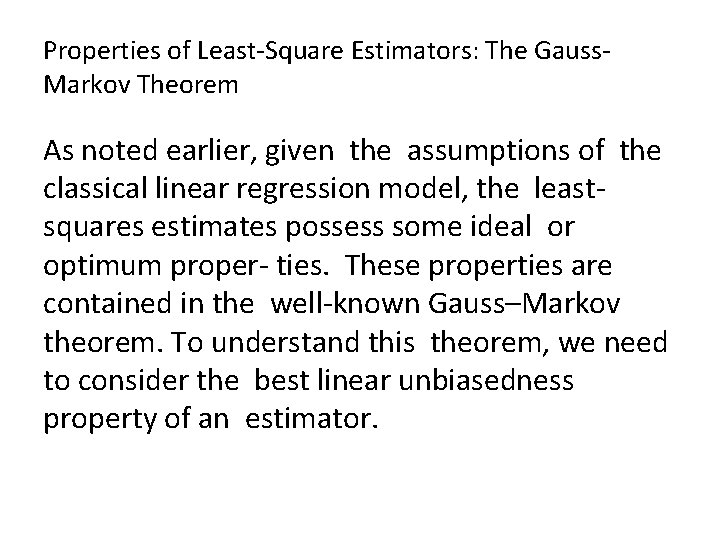Properties of Least-Square Estimators: The Gauss. Markov Theorem As noted earlier, given the assumptions of the classical linear regression model, the leastsquares estimates possess some ideal or optimum proper- ties. These properties are contained in the well-known Gauss–Markov theorem. To understand this theorem, we need to consider the best linear unbiasedness property of an estimator.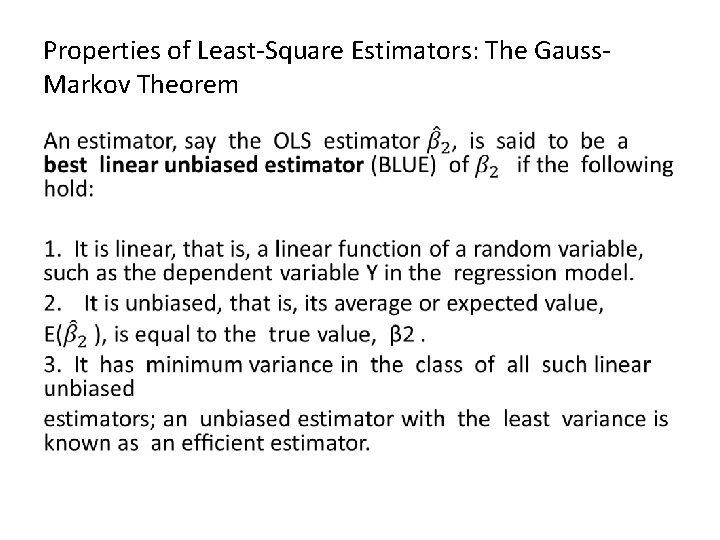Properties of Least-Square Estimators: The Gauss. Markov Theorem •